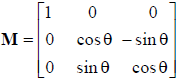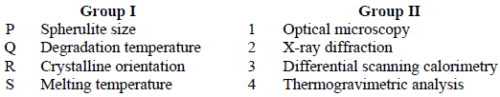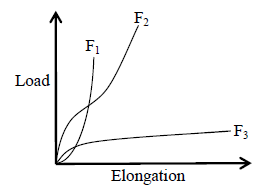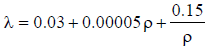# Textile Engineering And Fibre Science - TF 2012 GATE Paper (Practice Test)

## 65 Questions MCQ Test GATE Past Year Papers for Practice (All Branches) | Textile Engineering And Fibre Science - TF 2012 GATE Paper (Practice Test)

Description
Attempt Textile Engineering And Fibre Science - TF 2012 GATE Paper (Practice Test) | 65 questions in 180 minutes | Mock test for GATE preparation | Free important questions MCQ to study GATE Past Year Papers for Practice (All Branches) for GATE Exam | Download free PDF with solutions
QUESTION: 1

Solution:
QUESTION: 2

Solution:
QUESTION: 3

### Choose the grammatically CORRECT sentence:

Solution:
QUESTION: 4

Which one of the parts (A, B, C, D) in the sentence contains an ERROR?

No sooner had the doctor seen the results of the blood test, than he suggested the patient to see the specialist.

Solution:
QUESTION: 5

Ten teams participate in a tournament. Every team plays each of the other teams twice. The total number of matches to be played is

Solution:
QUESTION: 6

Q. 6 - Q. 10 carry two marks each.

Q. A value of x that satisfies the equation log x + log (x – 7) = log (x + 11) + log 2 is

Solution:
QUESTION: 7

Let f(x) = x – [x], where x ≥ 0 and [x] is the greatest integer not larger than x. Then f(x) is a

Solution:
QUESTION: 8

Ravi is taller than Arun but shorter than Iqbal. Sam is shorter than Ravi. Mohan is shorter than Arun. Balu is taller than Mohan and Sam. The tallest person can be

Solution:
QUESTION: 9

A smuggler has 10 capsules in which five are filled with narcotic drugs and the rest contain the original medicine. All the 10 capsules are mixed in a single box, from which the customs officials picked two capsules at random and tested for the presence of narcotic drugs. The probability that the smuggler will be caught is

Solution:
QUESTION: 10

The documents expose the cynicism of the government officials – and yet as the media website reflects, not a single newspaper has reported on their existence.

Which one of the following inferences may be drawn with the greatest accuracy from the above
passage?

Solution:
QUESTION: 11

Q. 11 – Q. 35 carry one mark each.

Q. The fibre which has a mineral origin is

Solution:
QUESTION: 12

The chemical that is used to convert soda cellulose to sodium cellulose xanthate in the manufacture of viscose rayon is

Solution:
QUESTION: 13

The fibre that will float on water is

Solution:
QUESTION: 14

The range of spinning speed (m/min) used in the manufacture of partially oriented polyester yarn is

Solution:
QUESTION: 15

Drawing of synthetic filament does not lead to an increase in

Solution:
QUESTION: 16

In a card, the wire point density is maximum on

Solution:
QUESTION: 17

The spinning system that does not generate false twist during spinning is

Solution:
QUESTION: 18

Most of the seed coat particles are removed in

Solution:
QUESTION: 19

An eccentric top roller in a drafting system leads to

Solution:
QUESTION: 20

The increase in traveller weight leads to an increase in

Solution:
QUESTION: 21

Dog knot is preferred to weaver's knot during creeling because it is

Solution:
QUESTION: 22

Size add-on does not depend on

Solution:
QUESTION: 23

Ball warping is mainly used in the manufacture of

Solution:
QUESTION: 24

The factor that does not influence the propelling force for moving the weft yarn on air jet loom is

Solution:
QUESTION: 25

In the context of thermal bonding of nonwoven web, the statement which is not true is

Solution:
QUESTION: 26

A 51 mm long fibre has 6 % crimp. The crimped length of the fibre in mm is approximately

Solution:
QUESTION: 27

On a mass based evenness tester, thin place in a yarn at −40 % setting is counted if mass per unit
length is

Solution:
QUESTION: 28

Ratio of grab strength to strip strength is the highest when fabric extension (%) is

Solution:
QUESTION: 29

Bursting strength of a woven fabric with the same warp and weft yarns is the highest when the ratio of ends/cm and picks/cm is

Solution:
QUESTION: 30

Fabric abrasion resistance cannot be assessed by the loss in

Solution:
QUESTION: 31

Bleached cotton fabric was sent to a laboratory for determination of Copper Number, which is an estimate of the presence of

Solution:
QUESTION: 32

Malachite Green is an important dyestuff. The typical green colour is obtained when the dye molecule is

Solution:
QUESTION: 33

A typical curve between equilibrium dye uptake and dyeing temperature goes through a maximum. After the maximum, the dye uptake decreases because

Solution:
QUESTION: 34

The efficacy of the wash-n-wear treatment can be estimated by measuring its

Solution:
QUESTION: 35

Softener reduces the bending rigidity of fabrics by decreasing

Solution:
*Answer can only contain numeric values
QUESTION: 36

Q. 36 to Q. 65 carry two marks each.

Questions Q.36 to Q. 40 are numerical answer type. The answer to each of these questions is either a positive whole number, or a positive real number with maximum of 2 decimal places.

Q. The density of a given polyester filament sample is 1.38 g/cm3. Consider the density of fully crystalline polyester as 1.455 g/cm3 and that of fully amorphous polyester as 1.335 g/cm3. The percent crystallinity of the sample is ________.

(Important - Enter only the numerical value in the answer)

Solution:
*Answer can only contain numeric values
QUESTION: 37

Cleaning efficiency of the opening roller in a rotor spinning machine, having 56 mm diameter rotor, is 80 %. The yarn breaks when the trash deposition within the rotor groove crosses the threshold of 1 mg/mm. If a sliver with 0.4 % trash is fed at 7.5 g/min, the number of end breaks in 1 hr (to the nearest whole number) is ________.

(Important - Enter only the numerical value in the answer)

Solution:
*Answer can only contain numeric values
QUESTION: 38

In a projectile weaving machine the projectile travels a total distance of 250 cm at an average velocity of 25 m/s. If the time period during which it is in motion occupies half of the loom cycle, the maximum loom speed in picks per minute is ________.

(Important - Enter only the numerical value in the answer)

Solution:
*Answer can only contain numeric values
QUESTION: 39

A 225 denier viscose yarn has a breaking strength of 7.5 N. The yarn tenacity in cN/dtex is ________.

(Important - Enter only the numerical value in the answer)

Solution:
*Answer can only contain numeric values
QUESTION: 40

In an experiment, 1 g each of the mercerized and unmercerized fabric samples are separately treated in 30 ml of 0.25N barium hydroxide solution for the required time. 10 ml of these solutions are drawn and titrated against 0.1N HCl solution. The volumes of HCl consumed at the end of these titrations are m for mercerized and u for unmercerized cases. If

Barium Activity Number (BAN) = [(b-m)/(b-u)]×100,

where m = 18 ml, u = 20 ml and b is the volume of HCl consumed in the blank titration, then the BAN value for the above mercerized sample is ________.

(Important - Enter only the numerical value in the answer)

Solution:
QUESTION: 41

Questions Q.41 to Q.65 are multiple choice type.

Q. Consider the following Assertion [a] and Reason [r]
[a] M is an orthogonal matrix, but not a skew-symmetric matrix.[r] Because MT = M−1 and MT ≠ −M.

Determine the correctness or otherwise of the above Assertion [a] and Reason [r]

Solution:
QUESTION: 42

A beaker contains 50 cc of an aqueous dye solution of concentration c (w/v). 25 cc of this solution
is removed and replaced by 25 cc of distilled water. This process is repeated five more times. The
final concentration of the solution is

Solution:
QUESTION: 43

Assume that the rate of evaporation of moisture from a wet fabric during drying process is proportional to the amount of moisture present in the fabric. If 50 % of the moisture is evaporated in the first 5 minutes then the time (min) taken to evaporate 90 % of the moisture is approximately

Solution:
QUESTION: 44

The number of neps in a carded web follows Poisson distribution with a mean of 100 per m2. The probability that there is no nep in an area of 645 cm2 is

Solution:
QUESTION: 45

A yarn of 24 mm length has a varying cross-section. The values of the cross-sectional area of yarn (mm2), measured at equal intervals of 4 mm from one end are

0.09, 0.12, 0.14, 0.15, 0.16, 0.13, 0.11

The volume of yarn (mm3) estimated by using Simpson's 1/3 rule of numerical integration is

Solution:
QUESTION: 46

Match the property from Group I with the characterization technique from Group II.Solution:
QUESTION: 47

Consider the following Assertion [a] and Reason [r]

[a] In the case of manufactured fibre spinning, a circular spinneret orifice always results in circular cross-section of filaments in melt spinning, but the same is not true in dry spinning.

[r] Melt spinning involves only heat transfer, whereas dry spinning involves heat as well as mass transfer.

Determine the correctness or otherwise of the above Assertion [a] and Reason [r]

Solution:
QUESTION: 48

Consider the following assertion [a] and reason [r] in the context of the load-elongation curves of fibres F1, F2, and F3[a] Fibre F3 is the most suitable fibre for making a rope for mountaineering.
[r] Mountaineering rope should have high tenacity, high modulus and high work of rupture.
Determine the correctness or otherwise of the above Assertion [a] and Reason [r]

Solution:
QUESTION: 49

The winding speed (difference between bobbin speed and traveller speed) of yarn in a ring frame is 200 rev/min when bobbin diameter is 28 mm. If the bobbin diameter is increased to 35 mm, the winding speed (rev/min) would be

Solution:
QUESTION: 50

The weight of material on a roving bobbin is 2.4 kg. The roving hank is 600 tex. If delivery rate is 20 m/min, the time (min) required to build the bobbin is

Solution:
QUESTION: 51

The terry towel fabric is a

Solution:
QUESTION: 52

Sizing of single cotton yarn leads to an increase in

Solution:
QUESTION: 53

The strength utilization of yarn in a woven fabric is

Solution:
QUESTION: 54

Yarns Y1 and Y2 have the same strength when tested at 200 mm gauge length. If mass unevenness CV (%) of yarns Y1 and Y2 are 10 and 20 respectively, the ratio of the strength of yarns Y1 and Ywhen tested at 500 mm gauge length is

Solution:
QUESTION: 55

Consider the following Assertion [a] and Reason [r]

[a] In the case of dyeing of cotton with reactive dyes, formation of hydrolyzed dye is a major problem.

[r] In an alkaline medium, the reactive dye reacts with the hydroxyl groups irrespective of whether these are from cellulose or water.

Determine the correctness or otherwise of the above Assertion [a] and Reason [r]

Solution:
QUESTION: 56

Consider the following Assertion [a] and Reason [r]

[a] In the case of durable press finishing of cotton fabrics, while the citric acid can be used as a crosslinking agent, oxalic acid cannot be.

[r] The mechanism of crosslinking requires formation of anhydride in the intermediate step.

Determine the correctness or otherwise of the above Assertion [a] and Reason [r]

Solution:
QUESTION: 57

Consider the following Assertion [a] and Reason [r]

[a] In the case of minimum application technique, compared to the conventional rolls of a typical
padding mangle, Roberto rolls substantially reduce the wet pick up.

[r] The Roberto rolls are connected to vacuum pump to facilitate removal of liquor from the fabric.

Determine the correctness or otherwise of the above Assertion [a] and Reason [r]

Solution:
QUESTION: 58

Common Data Questions

Common Data for Questions 58 and 59:

Four polyester and four cotton carded slivers of the same count and mass CV of 4.16 % are drawn together
keeping a draft of 8 on a breaker drawframe. Two slivers from breaker drawframe are further drawn along with the four cotton carded slivers keeping a draft of 6 on a finisher drawframe.

Q. The polyester (%) in the final sliver is approximately

Solution:
QUESTION: 59

Common Data Questions

Common Data for Questions 58 and 59:

Four polyester and four cotton carded slivers of the same count and mass CV of 4.16 % are drawn together
keeping a draft of 8 on a breaker drawframe. Two slivers from breaker drawframe are further drawn along with the four cotton carded slivers keeping a draft of 6 on a finisher drawframe.

Q. The mass CV (%) of the final sliver is approximately

Solution:
QUESTION: 60

Common Data for Questions 60 and 61:

A fabric is woven from 38 tex yarns of 0.65 packing coefficient and 1.54 g/cm3 fibre density. The fabric has 30 ends per cm and 25 picks per cm. Assume that warp is jammed.

Q. The weft crimp (%) is approximately

Solution:
QUESTION: 61

Common Data for Questions 60 and 61:

A fabric is woven from 38 tex yarns of 0.65 packing coefficient and 1.54 g/cm3 fibre density. The fabric
has 30 ends per cm and 25 picks per cm. Assume that warp is jammed.

Q. The thickness of the above fabric (mm) is approximately

Solution:
QUESTION: 62

The thermal conductivity of a nonwoven fabric is given bywhere λ is the thermal conductivity of the fabric in W/(m⋅K), ρ is the bulk density of the fabric in kg/m3,
and ρ ∈ [10,200].

Q. Thermal conductivity is minimum when the bulk density is approximately

Solution:
QUESTION: 63

The thermal conductivity of a nonwoven fabric is given bywhere λ is the thermal conductivity of the fabric in W/(m⋅K), ρ is the bulk density of the fabric in kg/m3,
and ρ ∈ [10,200].

Q. The minimum value of thermal conductivity, in mW/(m⋅K), is approximately

Solution:
QUESTION: 64

A cotton fibre of 180 millitex has a density of 1.5 g/cm3 and an average perimeter of 40 μm.

Q. The average area of the cell wall (μm2) is

Solution:
QUESTION: 65Use Code STAYHOME200 and get INR 200 additional OFF Use Coupon Code